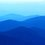# A discussion on an interesting number theory problem in RMO 2014

Problem :- Prove that there does not exist any integer n < 2310 such that n(2310-n) is a multiple of 2310.

I had written an alternative solution of this problem in the exam. Please go through the solution once and have your comments. Please inform about some improvements if necessary.

My solution :- We know that 2310 = 235711. So every prime factor in the prime factorization of 2310 occurs only once. So , suppose that n(2310-n) is divisible by 2310. Let p be a prime factor of 2310. So either p divides n or p divides 2310-n. Let us divide the problem into two cases :- Case 1:- p divides n :- If p divides n, then as p divides 2310, then p divides 2310-n. So n = pa and 2310-n = pb So n(2310-n) = (p^2)ab Hence (p^2) divides (2310-n)n Case 2 :- p divides 2310-n So, p must also divide n. Hence, in the same way, (p^2) again divides n(2310-n).

So we may conclude from both cases that if p divides 2310, then n(2310-n) is divisible by (p^2) So if 2310=abcd.....z where a......z are prime factors of 2310, then n(2310-n) = (a^2)(b^2)......(z^2)> (abc....z)^2 > (2310)^2 But we know that, n<2310 and 2310-n < 2310 So n(2310-n) < (2310)^2 So this leads to contradiction. Q.E.D.

Kindly comment on the solution.Note by Siddharth Kumar
6 years, 5 months ago

This discussion board is a place to discuss our Daily Challenges and the math and science related to those challenges. Explanations are more than just a solution — they should explain the steps and thinking strategies that you used to obtain the solution. Comments should further the discussion of math and science.

When posting on Brilliant:

• Use the emojis to react to an explanation, whether you're congratulating a job well done , or just really confused .
• Ask specific questions about the challenge or the steps in somebody's explanation. Well-posed questions can add a lot to the discussion, but posting "I don't understand!" doesn't help anyone.
• Try to contribute something new to the discussion, whether it is an extension, generalization or other idea related to the challenge.
• Stay on topic — we're all here to learn more about math and science, not to hear about your favorite get-rich-quick scheme or current world events.

MarkdownAppears as
*italics* or _italics_ italics
**bold** or __bold__ bold
- bulleted- list
• bulleted
• list
1. numbered2. list
1. numbered
2. list
Note: you must add a full line of space before and after lists for them to show up correctly
paragraph 1paragraph 2

paragraph 1

paragraph 2

[example link](https://brilliant.org)example link
> This is a quote
This is a quote
    # I indented these lines
# 4 spaces, and now they show
# up as a code block.

print "hello world"
# I indented these lines
# 4 spaces, and now they show
# up as a code block.

print "hello world"
MathAppears as
Remember to wrap math in $$ ... $$ or $ ... $ to ensure proper formatting.
2 \times 3 $2 \times 3$
2^{34} $2^{34}$
a_{i-1} $a_{i-1}$
\frac{2}{3} $\frac{2}{3}$
\sqrt{2} $\sqrt{2}$
\sum_{i=1}^3 $\sum_{i=1}^3$
\sin \theta $\sin \theta$
\boxed{123} $\boxed{123}$

## Comments

Sort by:

Top Newest

I think you don't have to divide the problem into two cases since the two cases are the same.

- 6 years, 5 months ago

Log in to reply

×

Problem Loading...

Note Loading...

Set Loading...